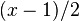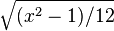# rn2

rn2(x)
Distribution uniform
Mean$(x-1)/2$
Standard deviation$\sqrt{(x^2-1)/12}$

rn2(x) is a pseudo-random number function used in the code of NetHack. The function's purpose is to provide an "unweighted": the range of values is between 0 and x-1 inclusive (that is, 0 ≤ rn2(x) < x).

For example, to simulate a percentage chance:

if (rn2(100) < chance) { ... }


Also,

!rn2(x)


is true with a 1/x probability.